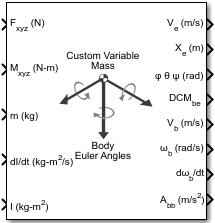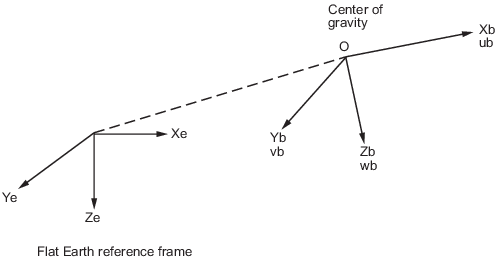Custom Variable Mass 6DOF (Euler Angles)

Implement Euler angle representation of six-degrees-of-freedom equations of motion of custom variable mass

• Library:
• Aerospace Blockset / Equations of Motion / 6DOF

•Description

The Custom Variable Mass 6DOF (Euler Angles) block implements the Euler angle representation of six-degrees-of-freedom equations of motion of custom variable mass. It considers the rotation of a body-fixed coordinate frame (Xb, Yb, Zb) about a flat Earth reference frame (Xe, Ye, Ze). For more information on Euler angles, see Algorithms.

Limitations

The block assumes that the applied forces act at the center of gravity of the body.

Ports

Input

expand all

Applied forces, specified as a three-element vector.

Data Types: double

Applied moments, specified as a three-element vector.

Data Types: double

One or more rates of change of mass (positive if accreted, negative if ablated), specified as a three-element vector.

Dependencies

To enable this port, select Include mass flow relative velocity.

Data Types: double

Mass, specified as a scalar.

Data Types: double

Rate of change of inertia tensor matrix, specified as a 3-by-3 matrix.

Data Types: double

Inertia tensor matrix, specified as a 3-by-3 matrix.

Data Types: double

One or more relative velocities at which the mass is accreted to or ablated from the body in body-fixed axes, specified as a three-element vector.

Dependencies

To enable this port, select Include mass flow relative velocity.

Data Types: double

Output

expand all

Velocity in the flat Earth reference frame, returned as a three-element vector.

Data Types: double

Position in the flat Earth reference frame, returned as a three-element vector.

Data Types: double

Euler rotation angles [roll, pitch, yaw], returned as a three-element vector, in radians.

Data Types: double

Coordinate transformation from flat Earth axes to body-fixed axes, returned as a 3-by-3 matrix.

Data Types: double

Velocity in body-fixed frame, returned as a three-element vector.

Data Types: double

Angular rates in body-fixed axes, returned as a three-element vector, in radians per second.

Data Types: double

Angular accelerations in body-fixed axes, returned as a three-element vector, in radians per second squared.

Data Types: double

Accelerations in body-fixed axes with respect to body frame, returned as a three-element vector.

Data Types: double

Accelerations in body-fixed axes with respect to inertial frame (flat Earth), returned as a three-element vector. You typically connect this signal to the accelerometer.

Dependencies

This port appears only when the Include inertial acceleration check box is selected.

Data Types: double

Parameters

expand all

Main

Input and output units, specified as Metric (MKS), English (Velocity in ft/s), or English (Velocity in kts).

UnitsForcesMomentAccelerationVelocityPositionMassInertia
Metric (MKS) NewtonNewton-meterMeters per second squaredMeters per secondMetersKilogramKilogram meter squared
English (Velocity in ft/s) PoundFoot-poundFeet per second squaredFeet per secondFeetSlugSlug foot squared
English (Velocity in kts) PoundFoot-poundFeet per second squaredKnotsFeetSlugSlug foot squared

Programmatic Use

 Block Parameter: units Type: character vector Values: Metric (MKS) | English (Velocity in ft/s) | English (Velocity in kts) Default: Metric (MKS)

Mass type, specified according to the following table.

Mass TypeDescriptionDefault for
Fixed

Mass is constant throughout the simulation.

Simple Variable

Mass and inertia vary linearly as a function of mass rate.

Custom Variable

Mass and inertia variations are customizable.

The Custom Variable selection conforms to the previously described equations of motion.

Programmatic Use

 Block Parameter: mtype Type: character vector Values: Fixed | Simple Variable | Custom Variable Default: 'Custom Variable'

Equations of motion representation, specified according to the following table.

RepresentationDescription

Euler Angles

Use Euler angles within equations of motion.

Quaternion

Use quaternions within equations of motion.

The Quaternion selection conforms to the equations of motion in Algorithms.

Programmatic Use

 Block Parameter: rep Type: character vector Values: Euler Angles | Quaternion Default: 'Euler Angles'

Initial location of the body in the flat Earth reference frame, specified as a three-element vector.

Programmatic Use

 Block Parameter: xme_0 Type: character vector Values: '[0 0 0]' | three-element vector Default: '[0 0 0]'

Initial velocity in body axes, specified as a three-element vector, in the body-fixed coordinate frame.

Programmatic Use

 Block Parameter: Vm_0 Type: character vector Values: '[0 0 0]' | three-element vector Default: '[0 0 0]'

Initial Euler orientation angles [roll, pitch, yaw], specified as a three-element vector, in radians. Euler rotation angles are those between the body and north-east-down (NED) coordinate systems.

Programmatic Use

 Block Parameter: eul_0 Type: character vector Values: '[0 0 0]' | three-element vector Default: '[0 0 0]'

Initial body-fixed angular rates with respect to the NED frame, specified as a three-element vector, in radians per second.

Programmatic Use

 Block Parameter: pm_0 Type: character vector Values: '[0 0 0]' | three-element vector Default: '[0 0 0]'

Select this check box to add a mass flow relative velocity port. This is the relative velocity at which the mass is accreted or ablated.

Programmatic Use

 Block Parameter: vre_flag Type: character vector Values: off | on Default: off

Select this check box to add an inertial acceleration port.

Dependencies

To enable the Abe port, select this parameter.

Programmatic Use

 Block Parameter: abi_flag Type: character vector Values: 'off' | 'on' Default: off

State Attributes

Assign a unique name to each state. You can use state names instead of block paths during linearization.

• To assign a name to a single state, enter a unique name between quotes, for example, 'velocity'.

• To assign names to multiple states, enter a comma-separated list surrounded by braces, for example, {'a', 'b', 'c'}. Each name must be unique.

• If a parameter is empty (' '), no name is assigned.

• The state names apply only to the selected block with the name parameter.

• The number of states must divide evenly among the number of state names.

• You can specify fewer names than states, but you cannot specify more names than states.

For example, you can specify two names in a system with four states. The first name applies to the first two states and the second name to the last two states.

• To assign state names with a variable in the MATLAB® workspace, enter the variable without quotes. A variable can be a character vector, cell array, or structure.

Position state names, specified as a comma-separated list surrounded by braces.

Programmatic Use

 Block Parameter: xme_statename Type: character vector Values: '' | comma-separated list surrounded by braces Default: ''

Velocity state names, specified as comma-separated list surrounded by braces.

Programmatic Use

 Block Parameter: Vm_statename Type: character vector Values: '' | comma-separated list surrounded by braces Default: ''

Euler rotation angle state names, specified as a comma-separated list surrounded by braces.

Programmatic Use

 Block Parameter: eul_statename Type: character vector Values: '' | comma-separated list surrounded by braces Default: ''

Body rotation rate state names, specified comma-separated list surrounded by braces.

Programmatic Use

 Block Parameter: pm_statename Type: character vector Values: '' | comma-separated list surrounded by braces Default: ''

Algorithms

The origin of the body-fixed coordinate frame is the center of gravity of the body. The body is assumed to be rigid, which eliminates the need to consider the forces acting between individual elements of mass. The flat Earth reference frame is considered inertial, an excellent approximation that allows the forces due to the Earth's motion relative to the “fixed stars” to be neglected.The translational motion of the body-fixed coordinate frame is given below, where the applied forces [Fx Fy Fz]T are in the body-fixed frame. Vreb is the relative velocity in the body axes at which the mass flow ($\stackrel{˙}{m}$) is ejected or added to the body-fixed axes.

$\begin{array}{l}{\overline{F}}_{b}=\left[\begin{array}{c}{F}_{x}\\ {F}_{y}\\ {F}_{z}\end{array}\right]=m\left({\stackrel{˙}{\overline{V}}}_{b}+\overline{\omega }×{\overline{V}}_{b}\right)+\stackrel{˙}{m}\overline{V}r{e}_{b}\\ {A}_{be}=\frac{{\overline{F}}_{b}-\stackrel{˙}{m}{\overline{V}}_{r{e}_{b}}}{m}\\ {A}_{bb}=\left[\begin{array}{c}{\stackrel{˙}{u}}_{b}\\ {\stackrel{˙}{v}}_{b}\\ {\stackrel{˙}{w}}_{b}\end{array}\right]=\frac{{\overline{F}}_{b}-\stackrel{˙}{m}{\overline{V}}_{r{e}_{b}}}{m}-\overline{\omega }×{\overline{V}}_{b}\\ {\overline{V}}_{b}=\left[\begin{array}{c}{u}_{b}\\ {v}_{b}\\ {w}_{b}\end{array}\right],\overline{\omega }=\left[\begin{array}{c}p\\ q\\ r\end{array}\right]\end{array}$

The rotational dynamics of the body-fixed frame are given below, where the applied moments are [L M N]T, and the inertia tensor I is with respect to the origin O.

$\begin{array}{l}{\overline{M}}_{B}=\left[\begin{array}{c}L\\ M\\ N\end{array}\right]=I\stackrel{˙}{\overline{\omega }}+\overline{\omega }×\left(I\overline{\omega }\right)+\stackrel{˙}{I}\overline{\omega }\\ \\ I=\left[\begin{array}{ccc}{I}_{xx}& -{I}_{xy}& -{I}_{xz}\\ -{I}_{yx}& {I}_{yy}& -{I}_{yz}\\ -{I}_{zx}& -{I}_{zy}& {I}_{zz}\end{array}\right]\\ \\ \stackrel{˙}{I}=\left[\begin{array}{ccc}{\stackrel{˙}{I}}_{xx}& -{\stackrel{˙}{I}}_{xy}& -{\stackrel{˙}{I}}_{xz}\\ -{\stackrel{˙}{I}}_{yx}& {\stackrel{˙}{I}}_{yy}& -{\stackrel{˙}{I}}_{yz}\\ -{\stackrel{˙}{I}}_{zx}& -{\stackrel{˙}{I}}_{zy}& {\stackrel{˙}{I}}_{zz}\end{array}\right]\end{array}$

The relationship between the body-fixed angular velocity vector, [p q r]T, and the rate of change of the Euler angles, ${\left[\stackrel{˙}{\varphi }\stackrel{˙}{\theta }\stackrel{˙}{\psi }\right]}^{\text{T}}$, can be determined by resolving the Euler rates into the body-fixed coordinate frame.

 $\left[\begin{array}{c}p\\ q\\ r\end{array}\right]=\left[\begin{array}{c}\stackrel{˙}{\varphi }\\ 0\\ 0\end{array}\right]+\left[\begin{array}{ccc}1& 0& 0\\ 0& \mathrm{cos}\varphi & \mathrm{sin}\varphi \\ 0& -\mathrm{sin}\varphi & \mathrm{cos}\varphi \end{array}\right]\left[\begin{array}{c}0\\ \stackrel{˙}{\theta }\\ 0\end{array}\right]+\left[\begin{array}{ccc}1& 0& 0\\ 0& \mathrm{cos}\varphi & \mathrm{sin}\varphi \\ 0& -\mathrm{sin}\varphi & \mathrm{cos}\varphi \end{array}\right]\left[\begin{array}{ccc}\mathrm{cos}\theta & 0& -\mathrm{sin}\theta \\ 0& 1& 0\\ \mathrm{sin}\theta & 0& \mathrm{cos}\theta \end{array}\right]\left[\begin{array}{c}0\\ 0\\ \stackrel{˙}{\psi }\end{array}\right]={J}^{-1}\left[\begin{array}{c}\stackrel{˙}{\varphi }\\ \stackrel{˙}{\theta }\\ \stackrel{˙}{\psi }\end{array}\right]$

Inverting J then gives the required relationship to determine the Euler rate vector.

$\left[\begin{array}{c}\stackrel{˙}{\varphi }\\ \stackrel{˙}{\theta }\\ \stackrel{˙}{\psi }\end{array}\right]=J\left[\begin{array}{c}p\\ q\\ r\end{array}\right]=\left[\begin{array}{ccc}1& \left(\mathrm{sin}\varphi \mathrm{tan}\theta \right)& \left(\mathrm{cos}\varphi \mathrm{tan}\theta \right)\\ 0& \mathrm{cos}\varphi & -\mathrm{sin}\varphi \\ 0& \frac{\mathrm{sin}\varphi }{\mathrm{cos}\theta }& \frac{\mathrm{cos}\varphi }{\mathrm{cos}\theta }\end{array}\right]\left[\begin{array}{c}p\\ q\\ r\end{array}\right]$